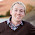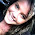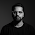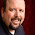## Tuesday, January 17, 2012

### TI-36X PRO: Programming Bug Info (VIDEO)In a previous post (link), I explained why the TI-36X PRO is a great calculator is you are taking the Fundamentals of Engineering or PE Exam. But it has one problem: a programming bug. Essentially, the bug is a display problem which only occurs when you try to display a mixed fraction involving pi, but still, any time your calculator is giving you wrong answers it is a serious problem.

I made a video to illustrate the bug, embedded below:

To check if your TI-36X PRO has the bug, first make sure you are in MathPrint mode. You can check this by pressing $$mode$$ and scrolling down to the bottom of the list of options. Now, you need to tell the calculator to display answers in mixed fraction form. To do this, type $$1.75$$ into he command line, then press $$math$$ then $$1$$ then enter. You should see the result $$1\frac{3}{4}$$. Then type $$\pi12.5^2$$ into the command line. When you hit $$enter$$, you should receive the answer $$490.8738521$$. Now press the answer toggle button (it is directly above $$enter$$) and your calculator will display $$154\frac{\pi}{4}$$ if your calculator has the bug. The mathematically correct answer would either be $$\pi154\frac{1}{4}$$ or $$\frac{\pi654}{4}$$. Remember to clear the memory (hit $$on$$ and $$clear$$ at the same time) after you try this!

Overall, I’m still a fan of the calculator. To avoid the bug, I never try to convert anything to a mixed fraction. There are very few times where I ever need a mixed fraction anyway. You can get all the information you need in either decimal or improper fraction form. I actually used this calculator on the Fundamentals of Engineering Exam and I was very happy with it.

LINKS:
TI-36 Wikipedia Page: http://en.wikipedia.org/wiki/TI-36#Programming_Errors
The Consumerist Article: http://consumerist.com/2011/09/this-texas-instruments-calculator-cant-calculate-correctly.html
Product Guidebook from TI: http://education.ti.com/downloads/guidebooks/scientific/36xPro/TI36PRO_Guidebook_EN.pdf
Here is a video from MrTechLabs describing the same problem:

#### 24 comments:

1.The answer is not wrong on the calculator it is telling you 156+pi/4 still just as it did with 1.75=1+3/4. This is not a bug it is just a misconception

1.No, 156+(pi/4)=156.785398... not 490.87385... which is the value we are trying to represent. The calculator's value is mathematically incorrect.

2.Okay, I'm a little confused. I just bought this calculator.. I did as you said to see if my calculator had the bug & the answer I got back was 156 π/4. Is this wrong? or right?

3.@rachel wrong indeed..the real value should be 490.8.jst think tis way,for solving (12.5^2)*pi ( 12.5^2 will be more than 144+ approximately ,which is multiplied by pi whose value is more than 3 so the real ans should be more than 450+ ie 490.8) our calculator is giving 156 pi/4=156.78..i hope ur confusion is nw cleared

4.The two correct answers should be pi*156-1/4 or (pi*625)/4

5.Oh, I get the problem. The calculator knows the number to be (156 and a fourth) of pi, but it displays 156 and pi over 4.

Mixed fractions are dumb anyway.

6.I just bought my TI36. I tried π12.5^2 and i got 154π/4. Then I hit ''on'' + ''clear''. Now after the memory cleared I'm getting 625π/4. Does it mean there is no more bug?

1.No. The bug is still there. You just have to refresh the bug by using math 1 at any time.

2.. If try to solve 5(-2) then it should give the answer of -10 .. but instead it gives me syntax error.. while casio calcualtor (991 es) gives correct answer.... help pelase?

3.@Hassaan: The TI-36X Pro uses different keys and symbols to indicate negative numbers (or exponents) and to indicate the subtraction operator. If you enter the wrong one you'll get a syntax error. Use the '(−)' key to enter a negative number (displayed with a slightly above center, shorter dash). Use the '−' key to enter the subtraction operator (displayed as a central, longer dash).

7.I too have gotten rid of the bug by holding down [on] and [clear] at the same time. Thanks!

8."On+Clear" Seems to have cleared up the issue for me as well... Strange...

9.Update on the TI36x Pro Bug

I am posting today to give everyone an update on the status of this problem. TI attempted a fix of this problem (sometime around Jan 2014) but the problem persists, but is nuanced a bit from the video, hence my post update.

My calculator has a manufacturing code of K-0614B which means it was assembled in June of 2014, and yes, my machine has this bug also, and YES it is a bug.

What 'triggers' the bug in the later level models of the TI36x Pro is the mixed number conversion algorithm [math]  [enter]. Once that happens EVEN once, the calculator will show incorrect results with PI in MathPrint. Again, clearing the memory (reseting the machine) clears the bug temporarily until the next mixed number conversion.

One, avoid mixed number conversions combined with PI. Two, be careful to clear the calculator after mixed number conversions if PI calcs are going to be subsequently performed. Three, you might want to set your MODE to engineering, and Classic. Remember, clearing the memory also sets your MODE to defaults!

OK, so let's see how this is nuanced on a 2014 TI36x Pro:
Clear the memory by holding [on] and pressing [clear], then press [clear].
Type [math]  [enter] (this will trigger the bug)
0 returns 0
Type PI 12.5 ^2 [<>] [enter]
156 PI/4 ouch, there is our bad result
(but now watch this, TI got half the fix right)
Type [<>] (to toggle back to decimal)
SYNTAX Error (whoa--- TI knows the form is bad!)

For those of you who might still be confused by this, by definition a mixed number may not contain a transcendental number in any of its parts; by definition the parts must all be whole integers. So, having PI as a numerator component of the fractional part of the mixed number is bogus; and this is still happening on recent deliverables from TI ONCE the mixed number conversion algorithm has been tripped.

I spoke with a rep from TI this morning and was told 'he' has never heard of it... he even asked me to walk him through the scenario, which I politely did. He told me he would report it ! ~nice, huh?

So, you have several options:
1) set the mode of your calculator in Classic, and Eng (three decimals) and leave it there; but, be aware that clearing the memory resets the defaults!
2) Avoid mixed number conversions with PI
3) Avoid mixed number conversions completely, and be careful to reset the machine if subsequent PI calcs are necessary.

The rep at TI said they DO NOT EVER recall calculators with bad logic. They DO replace or refund within the warranty period. He told me they would prefer I return it to the store if purchased within the first 30 days of the warranty period.

So, don't hold your breath for a fix, and never expect a recall--- having said all this, I'm not returning my calculator. It will probably be worth a lot of cash someday to calculator collectors (like me) because of this flaw--- depending on how many survive for the next thirty years! Beyond that, for the price, its still a fairly robust little machine!

Cheers folks!﻿

1.Mine has manufacturing code L-0315B (March 2015) and still behaves the same as yours.

2.Manufacturing code L-0515B (May 2015), same bug

3.Hi,
Can you confirm if the 4-way arrow key and the chrome operation keys have black marking?
I just bought this calculator with the manufacturing code K-0512A.
Mine does not have any and the keys are all chrome.
Are the newest models exactly same?

4.This bug still persists. Mine, manufactured Sep 2017 still has it. Not a big deal for me, as I don't think I'd ever use the mixed number conversion, but it's nice to know what the bug is.

10.There is another bug on this calculator that I have seen many times.

e.g. On num-solver, type in the equation 5=10+x and solve for x. Your answer will be x=-5, which is correct. Now here is the error, "when a previous num-solver gives you a negative value, like this one, it messes up your next num-solver."

Now that you have x=-5. Repeat the process using a different equation, such as x^2=12^2+20^2. This equation is also known as the pythagorean theorem. Your answer will come out -20, even though the answer should be 20. This happens with all types of equations. That was just an example.

Now, you're probably thinking, why not just change the "X value to 0" when solving for an equation, this will eliminate the negative value.

WELL.....Because that also gives errors.

For example. Try this equation 4.905x^2 = 17.68x when x=0 or even x=1. When you solve this equation, and many more!!! you get your new answer to be x=0. Which is wrong. However, if you change x to some other number like 2, you get x=3.6. Which is correct!!. This error happens when x=0 and x=1 and x=666666. And I"m sure it does it with more previous x values, I just haven't noticed it so far or even wasted my time trying to test it.

If anyone has a solution, please post it, because I find myself avoiding the num-solver all together.

CSUF student.

1.Keep in mind how the solvers work... an adroitly chosen X is used to sneak up on the correct value from one end or the other... and note also that X degree (2) equations have (two) solutions.
x=0 is one of the two solutions for 4.905x^2=17.68x; try using the poly-solv rather than num-solv. Also try putting the problem in standard form first/ 4.905x^2 - 17.68x + 0 = 0 now try using the poly-solv(1):
a=4.905
b= - 17.68
c=0 solve
x1=0
x2 = 3536 / 981 ~ 3.604485219

Try using num-solv only for linear (x degree 1) equations, and try using poly-solv for equations of (x degree 2 or 3).

Sometimes a small analysis is required to have an adroitly chosen X. Experiment a bit.

All in all, for \$20 bucks, this little gem from Kinpo (and TI) is pretty phenomenal.

Cheers folks,

2.Here is another example that will clarify how num-solv works.
3x^2 - 17x +3 =0
x1 ~= 0.18234
x2 ~= 5.48433 solved with poly-solv

Now, try with num-solv, using x=0 and then retry with x=7.

(remember, always press {ON} {CLEAR} to reset the calc including memories and modes before starting)
(alternately, try using 2nd {xyzt} to clear variables before beginning)

Now, try num-solv with x=7 for 3x^2 - 17x + 3 =0
x~=5.48433
(it found the 'right-most' value because solver snuck in from the right...)
Now, try num-solv with x=0 for 3x^2 - 17x + 3 =0
x~=0.18234
(this time solver found the 'left-most' value because our 'adroitly' chosen X facilitated sneaking in from the left...)

Both answers are of course correct because we are trying to find the solution to a second order equation which has two real roots. Go figure... or let solver figure it for you!

Cheers folks

11.The solution to this problem is simple...

Buy the Casio FX-115ES Plus / FX-991ES Plus C, or the Canon F-792SGA (the Canon is either based on the Casio FX-115ES Plus / FX-991ES Plus C, or the older non-"plus" versions. The Canon has improvements/features over the Casio, however, I'm not sure if it looses out on any features compared to the Casio).

There, simple fix :)

1.The FX-115 line of calcs is good, and like the TI 36X and 30X line of calcs (along with the HP33s HP35s) is also allowed on the FE exam... the Canon, however, is not (at least not yet).

Its really personal preference between the 36X Pro and the FX115. They're both very nice engineering tools and for non graphing non programable they are both rich in function and feature.

Personally, I have a substantial collection of calculators (most engineers do) and my preferences are HP35s, TI36XPro, FX115ES, TI84+Silver, and TI89Titanium.

Cheers folks,

12.. well I own this calc.. its great .. but their is a very big bug in it.... If try to solve 5(-2) then it should give the answer of -10 .. but instead it gives me syntax error.. while casio calcualtor (991 es) gives correct answer....

1.Should work fine if you are using the negative key(just checked on mine), but you will get an error if you incorrectly use the subtraction key.